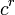# Linear representation theory of maximal unipotent subgroup of symplectic group of degree four over a finite field

## Contents

This article gives specific information, namely, linear representation theory, about a family of groups, namely: maximal unipotent subgroup of symplectic group of degree four. This article restricts attention to the case where the underlying ring is a finite field.
View linear representation theory of group families | View other specific information about maximal unipotent subgroup of symplectic group of degree four | View other specific information about group families for rings of the type finite field

Let$q$ be a prime power with underlying prime$p$ (we may have$q = p$). This page covers the linear representation theory of the maximal unipotent subgroup of the symplectic group of degree four$Sp(4,q)$ (in our naming convention, this stands for the group of$4 \times 4$ symplectic matrices) over the finite field of size$q$. This group can also be described as the$p$-Sylow subgroup of$Sp(4,q)$.

## Summary

Item Value
number of conjugacy classes (equals number of irreducible representations over a splitting field) Case$q$ even (i.e., a power of 2):$5q^2 - 6q + 2$
Case$q$ odd:$2q^2 - 1$
See number of irreducible representations equals number of conjugacy classes, element structure of maximal unipotent subgroup of symplectic group of degree four over a finite field
degrees of irreducible representations over a splitting field (such as$\overline{\mathbb{Q}}$ or$\mathbb{C}$) Case$q$ even (i.e., a power of 2): 1 (occurs$q^2$ times),$q/2$ (occurs$4(q - 1)^2$ times),$q$ (occurs$2(q - 1)$ times). NOTE: For$q = 2$,$1 = q/2$, so we have to count both types of occurences, and we get 1 (occurs$q^2 + 4(q-1)^2 = 8$ times), 2 (occurs 2 times)
Case$q$ odd: 1 (occurs$q^2$ times),$q$ (occurs$q^2 - 1$ times)
sum of squares of degrees of irreducible representations$q^4$ (equals order of the group)
see sum of squares of degrees of irreducible representations equals order of group
lcm of degrees of irreducible representations$q$
condition for a field (characteristic not equal to$p$) to be a splitting field The polynomial$x^p - 1$ should split completely.
For a finite field of size$s$, this is equivalent to$s \equiv 1 \pmod p$.
field generated by character values, which in this case also coincides with the unique minimal splitting field (characteristic zero) Field$\mathbb{Q}(\zeta)$ where$\zeta$ is a primitive$p^{th}$ root of unity. This is a degree$p - 1$ extension of the rationals.
unique minimal splitting field (characteristic$c \ne 0,p$) The field of size$c^r$ where$r$ is the order of$c$ mod$p$.

## Particular cases

Field size$q$ (a prime power) Field characteristic$p$$\log_pq$ Maximal unipotent subgroup of$Sp(4,q)$ Order of the group (equals$q^4$) Second part of GAP ID Number of irreducible representations equls number of conjugacy classes (equals$5q^2 - 6q + 2$ if$p = 2$, equals$2q^2 - 1$ otherwise) Degrees of irreducible representations (check against summary) Information on linear representation theory
2 2 1 direct product of D8 and Z2 16 11 10 1 (occurs 8 times), 2 (occurs 2 times) linear representation theory of direct product of D8 and Z2
3 3 1 wreath product of Z3 and Z3 81 7 17 1 (occurs 9 times), 3 (occurs 8 times) linear representation theory of wreath product of Z3 and Z3
4 2 2 free product of class two of two Klein four-groups 256 8935 58 1 (occurs 16 times), 2 (occurs 36 times), 4 (occurs 6 times) linear representation theory of free product of class two of two Klein four-groups
5 5 1 maximal unipotent subgroup of symplectic group:Sp(4,5) 625 7 49 1 (occurs 25 times), 5 (occurs 24 times) linear representation theory of maximal unipotent subgroup of symplectic group:Sp(4,5)